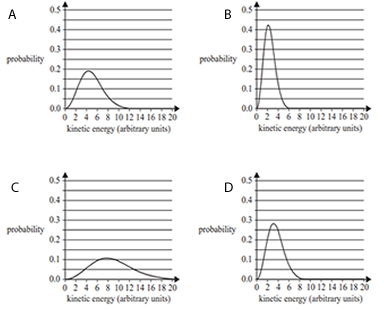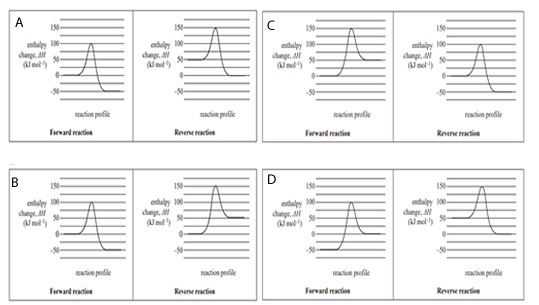Thermochemistry (2019 NHT) 1) 0.50 g of ethane, C2H6, undergoes complete combustion in a bomb calorimeter containing 200 mL of water. The water temperature rises from 22.0 °C to 48.5 °C. The thermochemical equation for the combustion of C2H6 using this information is A. C2H6 + 5O2 -> 2CO2 + 3H2O ΔH = –1330 kJ mol-1 B. 2C2H6 + 7O2 -> 4CO2 + 6H2O ΔH = –3120 kJ mol-1 C. C2H6 + 5O2 -> 2CO2 + 3H2O ΔH = –1560 kJ mol-1 D. 2C2H6 + 7O2 -> 4CO2 + 6H2O ΔH = –2660 kJ mol-1 Solution2) A student aims to calculate the theoretical amount of energy available to the body from cellular respiration using the oxygen gas, O2, retained by the body in a normal breath. In this calculation, the student assumes that: • the energy released at normal body temperature is the same as that released at standard laboratory conditions (SLC) • 19.6 mL of O2 is retained by the body in a normal breath. A balanced thermochemical equation for cellular respiration, with glucose as the primary reactant, is shown below. C6H12O6+ 6O2 -> 6CO2 + 6H2O ΔH = –2816 kJ mol-1 The theoretical amount of energy produced through cellular respiration from the O2 retained by the body in a normal breath would be A. 2.2 kJ B. 3.7 × 10-1 kJ C. 7.9 × 10-2 kJ D. 7.9 × 10-4 kJ Solution3) The Maxwell-Boltzmann distribution curves below represent the kinetic energies of molecules in a reaction with an activation energy, Ea , of 7 arbitrary units. Which one of the following shows the distribution curve with the highest proportion of successful collisions?Solution4) A reversible reaction has an enthalpy change, ΔH, of –50 kJ mol-1 for the forward reaction. Which one of the following pairs of energy profile diagrams, one for the forward reaction and one for the reverse reaction, represents this reaction?Solution5) Different types of fuels can be used in vehicles with combustion engines. These fuels can be produced from either fossil fuels or from renewable sources. Liquefied petroleum gas (LPG), a fossil fuel used in cars, is mainly made up of propane, C3H8. a. Write a balanced thermochemical equation for the complete combustion of C3H8 in air at standard laboratory conditions (SLC). Solutionb. How much energy, in kilojoules, would be produced from the complete combustion of 290 g of C3H8 at SLC? Solutionc. What volume of air (21.0% oxygen, O2, by volume), measured at SLC, would be required to fully combust 68.5 g of C3H8? Solution